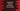# JavaScript program to check if an element is present in an Array or not## How to check if an element is present in an Array or not in `JavaScript`:

In this `JavaScript` example, we will learn how to check if an element is present in an `Array` or not in different ways. We can use a `for` loop or we can use the predefined array functions. Let me show you how it works with example programs.

### Method 1: By using a loop:

We can use a `for` or `while` loop to iterate over an array and find if an element is present in an Array or not. The following program shows how to use a `for` loop to check if a number is an array:

``````const isInArray = (arr, n) => {
for(let i = 0; i < arr.length; i++){
if(arr[i] === n) return true;
}
return false;
}

console.log(`2 is in [1, 2, 3, 4, 5, 6]: \${isInArray([1, 2, 3, 4, 5, 6], 2)}`);
console.log(`7 is in [1, 2, 3, 4, 5, 6]: \${isInArray([1, 2, 3, 4, 5, 6], 7)}`);``````

In this example, the `isInArray` method checks if the element `n` is in the array `arr` or not. It returns one `boolean` value. It iterates over the array element and compares each element with `n`. If you run the above program, it will print:

``````2 is in [1, 2, 3, 4, 5, 6]: true
7 is in [1, 2, 3, 4, 5, 6]: false``````

We can also use a `while` loop similar to the `for` loop.

### Method 2: With `indexOf`:

The `indexOf` method returns the first index of an element in an Array. If the element is not found in the array, it returns `-1`. The following program shows how to use the `indexOf` method to verify if an element is in an array:

``````const isInArray = (arr, n) => {
return arr.indexOf(n) !== -1;
}

console.log(`2 is in [1, 2, 3, 4, 5, 6]: \${isInArray([1, 2, 3, 4, 5, 6], 2)}`);
console.log(`7 is in [1, 2, 3, 4, 5, 6]: \${isInArray([1, 2, 3, 4, 5, 6], 7)}`);``````

It will print the same output.

``````2 is in [1, 2, 3, 4, 5, 6]: true
7 is in [1, 2, 3, 4, 5, 6]: false``````

It finds the index of `n` in the array `arr` and returns `false` if the result of `indexOf` is `-1`. Else, it returns `true`.

### Method 3: By using the `includes` method:

The `includes()` method checks if an element is included in an array or not and returns one boolean value. It returns `true` if the element is in the array. Else, it returns `false`.

The syntax of the `includes()` method is:

``````includes(e)
includes(e, from)``````

Where,

• The parameter `e` is the element to search in the array.
• The second parameter `from` is an optional parameter. This is the index to start the search. We can also use negative index for `from`. It will count back from the array end. It will use `array-length + from` if `from < 0`.
• If the value of `from` is greater than or equal to the length of the array, it will return `false`. If its value is smaller than `-array.length`, it will search the entire array.

The `includes` method returns a boolean value. It returns `true` if the element is found.

Let me modify the above example to use the `includes` method to check if an element is in an array or not:

``````const isInArray = (arr, n) => {
return arr.includes(n);
}

console.log(`2 is in [1, 2, 3, 4, 5, 6]: \${isInArray([1, 2, 3, 4, 5, 6], 2)}`);
console.log(`7 is in [1, 2, 3, 4, 5, 6]: \${isInArray([1, 2, 3, 4, 5, 6], 7)}`);``````

It will print similar output.

#### How to use the `includes` method to start the search from a specific index:

We can use the `includes` method with the second parameter to search from a specific index. For example,

``````const isInArray = (arr, n, from) => {
return arr.includes(n, from);
}

const arr = [1, 2, 3, 4, 5, 6, 7, 8, 9, 10];

console.log(`Search for 3 starting index 3: \${isInArray(arr, 3, 3)}`);
console.log(`Search for 3 starting index 1: \${isInArray(arr, 3, 1)}`);``````

One new parameter, `from` is added to the `isInArray` method. This parameter is passed as the second parameter to the `includes` method.

The first one is starting the search from index 3 and the second example is starting the search from index 1. Since the index starts at 0, the first example will print `false` and the second example will print `true`.

``````Search for 3 starting index 3: false
Search for 3 starting index 1: true``````